## The F-Test

An F-test is any statistical test in which the test statistic has an F-distribution under the null hypothesis.

### Learning Objectives

Summarize the F-statistic, the F-test and the F-distribution.

### Key Takeaways

#### Key Points

• The F-test is most often used when comparing statistical models that have been fitted to a data set, in order to identify the model that best fits the population from which the data were sampled.
• Perhaps the most common F-test is that which tests the hypothesis that the means and standard deviations of several populations are equal. (Note that all populations involved must be assumed to be normally distributed.)
• The F-test is sensitive to non-normality.
• The F-distribution is skewed to the right, but as the degrees of freedom for the numerator and for the denominator get larger, the curve approximates the normal.

#### Key Terms

• ANOVA: Analysis of variance—a collection of statistical models used to analyze the differences between group means and their associated procedures (such as “variation” among and between groups).
• Type I error: Rejecting the null hypothesis when the null hypothesis is true.
• F-Test: A statistical test using the F-distribution, most often used when comparing statistical models that have been fitted to a data set, in order to identify the model that best fits the population from which the data were sampled.

An F-test is any statistical test in which the test statistic has an F-distribution under the null hypothesis. It is most often used when comparing statistical models that have been fitted to a data set, in order to identify the model that best fits the population from which the data were sampled. Exact F-tests mainly arise when the models have been fitted to the data using least squares. The name was coined by George W. Snedecor, in honour of Sir Ronald A. Fisher. Fisher initially developed the statistic as the variance ratio in the 1920s.

The F-test is sensitive to non-normality. In the analysis of variance (ANOVA), alternative tests include Levene’s test, Bartlett’s test, and the Brown–Forsythe test. However, when any of these tests are conducted to test the underlying assumption of homoscedasticity (i.e., homogeneity of variance), as a preliminary step to testing for mean effects, there is an increase in the experiment-wise type I error rate.

Examples of F-tests include:

1. The hypothesis that the means and standard deviations of several populations are equal. (Note that all populations involved must be assumed to be normally distributed.) This is perhaps the best-known F-test, and plays an important role in the analysis of variance (ANOVA).
2. The hypothesis that a proposed regression model fits the data well (lack-of-fit sum of squares).
3. The hypothesis that a data set in a regression analysis follows the simpler of two proposed linear models that are nested within each other.
4. Scheffé’s method for multiple comparisons adjustment in linear models.

### The F-Distribution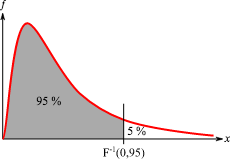F-distribution: The F-distribution is skewed to the right and begins at the x-axis, meaning that F-values are always positive.

The F-distribution exhibits the following properties, as illustrated in the above graph:

1. The curve is not symmetrical but is skewed to the right.
2. There is a different curve for each set of degrees of freedom.
3. The F-statistic is greater than or equal to zero.
4. As the degrees of freedom for the numerator and for the denominator get larger, the curve approximates the normal.

The F-statistic also has a common table of values, as do zscores and t-scores.

## The One-Way F-Test

The $\text{F}$-test as a one-way analysis of variance assesses whether the expected values of a quantitative variable within groups differ from each other.

### Learning Objectives

Explain the purpose of the one-way ANOVA $\text{F}$-test and perform the necessary calculations.

### Key Takeaways

#### Key Points

• The advantage of the ANOVA $\text{F}$-test is that we do not need to pre-specify which treatments are to be compared, and we do not need to adjust for making multiple comparisons.
• The disadvantage of the ANOVA $\text{F}$-test is that if we reject the null hypothesis, we do not know which treatments can be said to be significantly different from the others.
• If the $\text{F}$-test is performed at level $\alpha$ we cannot state that the treatment pair with the greatest mean difference is significantly different at level $\alpha$.
• The $\text{F}$– statistic will be large if the between-group variability is large relative to the within-group variability, which is unlikely to happen if the population means of the groups all have the same value.

#### Key Terms

• omnibus: containing multiple items
• F-Test: a statistical test using the $\text{F}$ distribution, most often used when comparing statistical models that have been fitted to a data set, in order to identify the model that best fits the population from which the data were sampled
• ANOVA: Analysis of variance—a collection of statistical models used to analyze the differences between group means and their associated procedures (such as “variation” among and between groups).

The $\text{F}$ test as a one-way analysis of variance is used to assess whether the expected values of a quantitative variable within several pre-defined groups differ from each other. For example, suppose that a medical trial compares four treatments. The ANOVA $\text{F}$-test can be used to assess whether any of the treatments is on average superior, or inferior, to the others versus the null hypothesis that all four treatments yield the same mean response. This is an example of an “omnibus” test, meaning that a single test is performed to detect any of several possible differences.

Alternatively, we could carry out pairwise tests among the treatments (for instance, in the medical trial example with four treatments we could carry out six tests among pairs of treatments). The advantage of the ANOVA $\text{F}$-test is that we do not need to pre-specify which treatments are to be compared, and we do not need to adjust for making multiple comparisons. The disadvantage of the ANOVA $\text{F}$-test is that if we reject the null hypothesis, we do not know which treatments can be said to be significantly different from the others. If the $\text{F}$-test is performed at level $\alpha$ we cannot state that the treatment pair with the greatest mean difference is significantly different at level $\alpha$.

The formula for the one-way ANOVA $\text{F}$-test statistic is:

$\text{F}=\dfrac { \text{explained variance} }{ \text{unexplained variance} }$

or

$\text{F}=\dfrac { \text{between-group variability} }{ \text{within-group variability} }$

The “explained variance,” or “between-group variability” is:

$\displaystyle \sum _{ \text{i} }^{ } \frac{{ \text{n} }_{ \text{i} }{ \left( { \bar { \text{Y} } }_{ \text{i} }-\bar { \text{Y} } \right) }^{ 2 }}{\left( \text{K}-1 \right)}$

where ${ \bar { \text{Y} } }_{ \text{i} }$ denotes the sample mean in the $\text{i}$th group, $\text{n}_\text{i}$ is the number of observations in the $\text{i}$th group, $\bar { \text{Y} }$ denotes the overall mean of the data, and $\text{K}$ denotes the number of groups.

The “unexplained variance”, or “within-group variability” is:

$\displaystyle \sum _{ \text{ij} }^{ }\frac{{ \left( { \bar { \text{Y} } }_{ \text{ij} }-{ \bar { \text{Y} } }_{ \text{i} } \right) }^{ 2 }} {\left( \text{N}-\text{K} \right)}$

where $\bar{\text{Y}_{\text{ij}}}$ is the $\text{j}$th observation in the $\text{i}$th out of $\text{K}$ groups and $\text{N}$ is the overall sample size. This $\text{F}$-statistic follows the $\text{F}$– distribution with $\text{K}-1$, $\text{N}-\text{K}$ degrees of freedom under the null hypothesis. The statistic will be large if the between-group variability is large relative to the within-group variability, which is unlikely to happen if the population means of the groups all have the same value.

Note that when there are only two groups for the one-way ANOVA $\text{F}$-test, $\text{F}=\text{t}^2$ where $\text{t}$ is the Student’s $\text{t}$-statistic.

### Example

Four sororities took a random sample of sisters regarding their grade means for the past term. The data were distributed as follows:

• Sorority 1: 2.17, 1.85, 2.83, 1.69, 3.33
• Sorority 2: 2.63,1.77, 3.25, 1.86, 2.21
• Sorority 3: 2.63, 3.78, 4.00, 2.55, 2.45
• Sorority 4: 3.79, 3.45, 3.08, 2.26, 3.18

Using a significance level of 1%, is there a difference in mean grades among the sororities?

#### Solution

Let $\mu_1$, $\mu_2$, $\mu_3$, $\mu_4$ be the population means of the sororities. Remember that the null hypothesis claims that the sorority groups are from the same normal distribution. The alternate hypothesis says that at least two of the sorority groups come from populations with different normal distributions. Notice that the four sample sizes are each size 5. Also, note that this is an example of a balanced design, since each factor (i.e., sorority) has the same number of observations.

$\text{H}_0: \mu_1 = \mu_2 = \mu_3 = \mu_4$

$\text{H}_\text{a}:$ Not all of the means $\mu_1$, $\mu_2$, $\mu_3$, $\mu_4$ are equal

Distribution for the test: $\text{F}_{3, 16}$

where $\text{k}=4$ groups and $\text{n}=20$ samples in total

$\text{df}_{\text{numerator}} = \text{k}-1 = 4-1 = 3$

$\text{df}_{\text{denominator}} = \text{n}-\text{k} = 20-4 = 16$

Calculate the test statistic: $\text{F}=2.23$

Graph: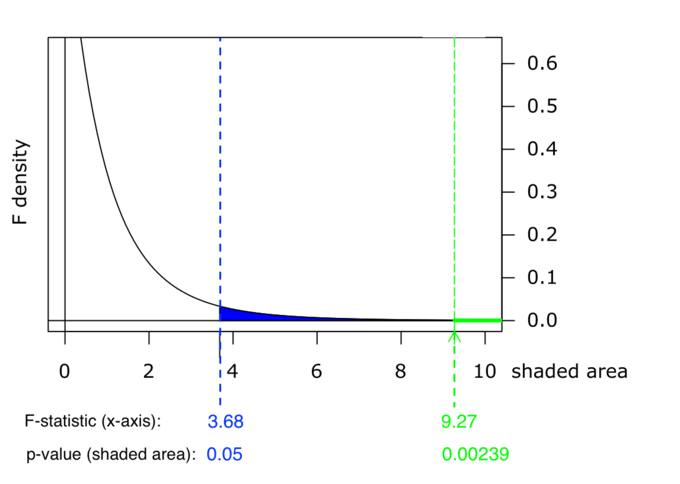Graph of $\text{p}$-Value: This chart shows example p-values for two F-statistics: p = 0.05 for F = 3.68, and p = 0.00239 for F = 9.27. These numbers are evidence of the skewness of the F-curve to the right; a much higher F-value corresponds to an only slightly smaller p-value.

Probability statement: $\text{p}\text{-value} = \text{P}(\text{F}>2.23) = 0.1241$

Compare $\alpha$ and the $\text{p}$-value: $\alpha = 0.01$, $\text{p}\text{-value} = 0.1241$

Make a decision: Since $\alpha < \text{p}\text{-value}$, you cannot reject $\text{H}_0$.

Conclusion: There is not sufficient evidence to conclude that there is a difference among the mean grades for the sororities.

## Variance Estimates

The $\text{F}$-test can be used to test the hypothesis that the variances of two populations are equal.

### Learning Objectives

Discuss the $\text{F}$-test for equality of variances, its method, and its properties.

### Key Takeaways

#### Key Points

• This $\text{F}$-test needs to be used with caution, as it can be especially sensitive to the assumption that the variables have a normal distribution.
• This test is of importance in mathematical statistics, since it provides a basic exemplar case in which the $\text{F}$-distribution can be derived.
• The null hypothesis is rejected if $\text{F}$ is either too large or too small.
• $\text{F}$-tests are used for other statistical tests of hypotheses, such as testing for differences in means in three or more groups, or in factorial layouts.

#### Key Terms

• F-Test: A statistical test using the $\text{F}$ distribution, most often used when comparing statistical models that have been fitted to a data set, in order to identify the model that best fits the population from which the data were sampled.
• variance: a measure of how far a set of numbers is spread out

### $\text{F}$-Test of Equality of Variances

An $\text{F}$-test for the null hypothesis that two normal populations have the same variance is sometimes used; although, it needs to be used with caution as it can be sensitive to the assumption that the variables have this distribution.

Notionally, any $\text{F}$-test can be regarded as a comparison of two variances, but the specific case being discussed here is that of two populations, where the test statistic used is the ratio of two sample variances. This particular situation is of importance in mathematical statistics since it provides a basic exemplar case in which the $\text{F}$ distribution can be derived.

### The Test

Let $\text{X}_1, \dots, \text{X}_\text{n}$ and $\text{Y}_1, \dots, \text{Y}_\text{m}$ be independent and identically distributed samples from two populations which each have a normal distribution. The expected values for the two populations can be different, and the hypothesis to be tested is that the variances are equal. The test statistic is:

$\displaystyle \text{F} = \frac{\text{S}^{2}_{\text{X}}}{\text{S}^{2}_{\text{Y}}}$

It has an $\text{F}$-distribution with $\text{n}-1$ and $\text{m}-1$ degrees of freedom if the null hypothesis of equality of variances is true. The null hypothesis is rejected if $\text{F}$ is either too large or too small. The immediate assumption of the problem outlined above is that it is a situation in which there are more than two groups or populations, and the hypothesis is that all of the variances are equal.

### Properties of the $\text{F}$ Test

This $\text{F}$-test is known to be extremely sensitive to non-normality. Therefore, they must be used with care, and they must be subject to associated diagnostic checking.

$\text{F}$-tests are used for other statistical tests of hypotheses, such as testing for differences in means in three or more groups, or in factorial layouts. These $\text{F}$-tests are generally not robust when there are violations of the assumption that each population follows the normal distribution, particularly for small alpha levels and unbalanced layouts. However, for large alpha levels (e.g., at least 0.05) and balanced layouts, the $\text{F}$-test is relatively robust. Although, if the normality assumption does not hold, it suffers from a loss in comparative statistical power as compared with non-parametric counterparts.

## Mean Squares and the F-Ratio

Most $\text{F}$-tests arise by considering a decomposition of the variability in a collection of data in terms of sums of squares.

### Learning Objectives

Demonstrate how sums of squares and mean squares produce the $\text{F}$-ratio and the implications that changes in mean squares have on it.

### Key Takeaways

#### Key Points

• The test statistic in an $\text{F}$-test is the ratio of two scaled sums of squares reflecting different sources of variability.
• These sums of squares are constructed so that the statistic tends to be greater when the null hypothesis is not true.
• To calculate the $\text{F}$-ratio, two estimates of the variance are made: variance between samples and variance within samples.
• The one-way ANOVA test depends on the fact that the mean squares between samples can be influenced by population differences among means of the several groups.

#### Key Terms

• pooled variance: A method for estimating variance given several different samples taken in different circumstances where the mean may vary between samples but the true variance is assumed to remain the same.
• null hypothesis: A hypothesis set up to be refuted in order to support an alternative hypothesis; presumed true until statistical evidence in the form of a hypothesis test indicates otherwise.

Most $\text{F}$-tests arise by considering a decomposition of the variability in a collection of data in terms of sums of squares. The test statistic in an $\text{F}$-test is the ratio of two scaled sums of squares reflecting different sources of variability. These sums of squares are constructed so that the statistic tends to be greater when the null hypothesis is not true. In order for the statistic to follow the $\text{F}$– distribution under the null hypothesis, the sums of squares should be statistically independent, and each should follow a scaled chi-squared distribution. The latter condition is guaranteed if the data values are independent and normally distributed with a common variance.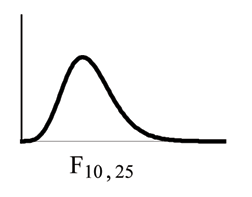$\text{F}$-Distribution: The $\text{F}$ ratio follows the $\text{F}$-distribution, which is right skewed.

There are two sets of degrees of freedom for the $\text{F}$-ratio: one for the numerator and one for the denominator. For example, if $\text{F}$ follows an $\text{F}$-distribution and the degrees of freedom for the numerator are 4 and the degrees of freedom for the denominator are 10, then $\text{F} \sim \text{F}_{4, 10}$.

To calculate the $\text{F}$-ratio, two estimates of the variance are made:

1. Variance between samples: An estimate of $\sigma^2$ that is the variance of the sample means multiplied by $\text{n}$ (when there is equal $\text{n}$). If the samples are different sizes, the variance between samples is weighted to account for the different sample sizes. The variance is also called variation due to treatment or explained variation.
2. Variance within samples: An estimate of $\sigma^2$ that is the average of the sample variances (also known as a pooled variance). When the sample sizes are different, the variance within samples is weighted. The variance is also called the variation due to error or unexplained variation.
• $\text{SS}_{\text{between}}$ is the sum of squares that represents the variation among the different samples.
• $\text{SS}_{\text{within}}$ is the sum of squares that represents the variation within samples that is due to chance.

To find a “sum of squares” is to add together squared quantities which, in some cases, may be weighted. $\text{MS}$ means “mean square. ” $\text{MS}_{\text{between}}$ is the variance between groups and $\text{MS}_{\text{within}}$ is the variance within groups.

### Calculation of Sum of Squares and Mean Square

• $\text{k}$ is the number of different groups
• $\text{n}_\text{j}$ is the size of the $\text{j}$th group
• $\text{s}_\text{j}$ is the sum of the values in the $\text{j}$th group
• $\text{n}$ is total number of all the values combined. (Total sample size: $\sum_\text{j} \text{n}_\text{j}$)
• $\text{x}$ is one value: $\sum \text{x} = \sum_\text{j} \text{s}_\text{j}$
• Sum of squares of all values from every group combined: $\sum \text{x}^2$
• Between group variability: $\displaystyle { \text{SS} }_{ \text{total} }=\sum { { \text{x} }^{ 2 }- } \frac { { \left( \sum { \text{x} } \right) }^{ 2 } }{ n }$
• Total sum of squares: $\displaystyle \sum { { \text{x} }^{ 2 }- } \frac { { \left( \sum { \text{x} } \right) }^{ 2 } }{ \text{n} }$
• Explained variation: sum of squares representing variation among the different samples $\displaystyle { \text{SS} }_{ \text{between} }=\sum { \left[ \frac { { \left( \text{s}_\text{j} \right) }^{ 2 } }{ { \text{n} }_{ \text{j} } } \right] - } \frac { { \left( \sum { { \text{s} }_{ \text{j} } } \right) }^{ 2 } }{ \text{n} }$
• Unexplained variation: sum of squares representing variation within samples due to chance: $\text{SS}_{\text{within}} = \text{SS}_{\text{total}} = \text{SS}_{\text{between}}$
• $\text{df}$‘s for different groups ($\text{df}$‘s for the numerator): $\text{df}_{\text{between}} = \text{k}-1$
• Equation for errors within samples ($\text{df}$‘s for the denominator): $\text{df}_{\text{within}} = \text{n}-\text{k}$
• Mean square (variance estimate) explained by the different groups: $\displaystyle { \text{MS} }_{ \text{between} }=\frac { { \text{SS} }_{ \text{between} } }{ { \text{df} }_{ \text{between} } }$
• Mean square (variance estimate) that is due to chance (unexplained): $\displaystyle{ \text{MS} }_{ \text{within} }=\frac { { \text{SS} }_{ \text{within} } }{ { \text{df} }_{ \text{within} } }$

MSbetween and MSwithin can be written as follows:

• $\displaystyle { \text{MS} }_{ \text{between} }=\frac { { \text{SS} }_{ \text{between} } }{ { \text{df} }_{ \text{between} } } =\frac { { \text{SS} }_{ \text{between} } }{ \text{k}-1 }$
• $\displaystyle { \text{MS} }_{ \text{within} }=\frac { { \text{SS} }_{ \text{within} } }{ { \text{df} }_{ \text{within} } } =\frac { { \text{SS} }_{ \text{within} } }{ \text{n}-\text{k} }$

The one-way ANOVA test depends on the fact that $\text{MS}_{\text{between}}$ can be influenced by population differences among means of the several groups. Since $\text{MS}_{\text{within}}$ compares values of each group to its own group mean, the fact that group means might be different does not affect $\text{MS}_{\text{within}}$.

The null hypothesis says that all groups are samples from populations having the same normal distribution. The alternate hypothesis says that at least two of the sample groups come from populations with different normal distributions. If the null hypothesis is true, $\text{MS}_{\text{between}}$ and $\text{MS}_{\text{within}}$ should both estimate the same value. Note that the null hypothesis says that all the group population means are equal. The hypothesis of equal means implies that the populations have the same normal distribution because it is assumed that the populations are normal and that they have equal variances.

### F Ratio

$\displaystyle \text{F}=\frac { { \text{MS} }_{ \text{between} } }{ { \text{MS} }_{ \text{within} } }$

If $\text{MS}_{\text{between}}$ and $\text{MS}_{\text{within}}$ estimate the same value (following the belief that Ho is true), then the F-ratio should be approximately equal to one. Mostly just sampling errors would contribute to variations away from one. As it turns out, $\text{MS}_{\text{between}}$ consists of the population variance plus a variance produced from the differences between the samples. $\text{MS}_{\text{within}}$ is an estimate of the population variance. Since variances are always positive, if the null hypothesis is false, $\text{MS}_{\text{between}}$ will generally be larger than $\text{MS}_{\text{within}}$. Then, the F-ratio will be larger than one. However, if the population effect size is small it is not unlikely that $\text{MS}_{\text{within}}$ will be larger in a give sample.

## ANOVA

ANOVA is a statistical tool used in several ways to develop and confirm an explanation for the observed data.

### Learning Objectives

Recognize how ANOVA allows us to test variables in three or more groups.

### Key Takeaways

#### Key Points

• ANOVA is a particular form of statistical hypothesis testing heavily used in the analysis of experimental data.
• ANOVA is used in the analysis of comparative experiments —those in which only the difference in outcomes is of interest.
• The statistical significance of the experiment is determined by a ratio of two variances.
• The calculations of ANOVA can be characterized as computing a number of means and variances, dividing two variances and comparing the ratio to a handbook value to determine statistical significance.
• ANOVA statistical significance results are independent of constant bias and scaling errors as well as the units used in expressing observations.

#### Key Terms

• ANOVA: Analysis of variance—a collection of statistical models used to analyze the differences between group means and their associated procedures (such as “variation” among and between groups).
• null hypothesis: A hypothesis set up to be refuted in order to support an alternative hypothesis; presumed true until statistical evidence in the form of a hypothesis test indicates otherwise.

Many statistical applications in psychology, social science, business administration, and the natural sciences involve several groups. For example, an environmentalist is interested in knowing if the average amount of pollution varies in several bodies of water. A sociologist is interested in knowing if the amount of income a person earns varies according to his or her upbringing. A consumer looking for a new car might compare the average gas mileage of several models. For hypothesis tests involving more than two averages, statisticians have developed a method called analysis of variance (abbreviated ANOVA).

ANOVA is a collection of statistical models used to analyze the differences between group means and their associated procedures (such as “variation” among and between groups). In ANOVA setting, the observed variance in a particular variable is partitioned into components attributable to different sources of variation. In its simplest form, ANOVA provides a statistical test of whether or not the means of several groups are equal, and therefore generalizes t-test to more than two groups. Doing multiple two- sample t-tests would result in an increased chance of committing a type I error. For this reason, ANOVAs are useful in comparing (testing) three or more means (groups or variables) for statistical significance.

ANOVA is a particular form of statistical hypothesis testing heavily used in the analysis of experimental data. In the typical application of ANOVA, the null hypothesis is that all groups are simply random samples of the same population. This implies that all treatments have the same effect (perhaps none). Rejecting the null hypothesis implies that different treatments result in altered effects.

### Characteristics of ANOVA

ANOVA is used in the analysis of comparative experiments—those in which only the difference in outcomes is of interest. The statistical significance of the experiment is determined by a ratio of two variances. This ratio is independent of several possible alterations to the experimental observations, so that adding a constant to all observations, or multiplying all observations by a constant, does not alter significance. Therefore, ANOVA statistical significance results are independent of constant bias and scaling errors as well as the units used in expressing observations.

The calculations of ANOVA can be characterized as computing a number of means and variances, dividing two variances and comparing the ratio to a handbook value to determine statistical significance. Calculating a treatment effect is then trivial; therefore, the effect of any treatment is estimated by taking the difference between the mean of the observations which receive the treatment and the general mean.

### Summary

ANOVA is the synthesis of several ideas and it is used for multiple purposes. As a consequence, it is difficult to define concisely or precisely. In short, ANOVA is a statistical tool used in several ways to develop and confirm an explanation for the observed data. Additionally:

1. It is computationally elegant and relatively robust against violations to its assumptions.
2. ANOVA provides industrial strength (multiple sample comparison) statistical analysis.
3. It has been adapted to the analysis of a variety of experimental designs.

As a result, ANOVA has long enjoyed the status of being the most used (some would say abused) statistical technique in psychological research, and ANOVA is probably the most useful technique in the field of statistical inference. ANOVA with a very good fit and ANOVA with no fit are shown, respectively, in and.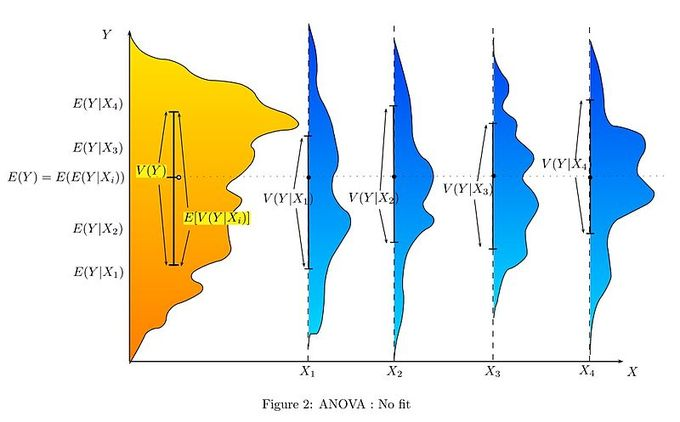ANOVA With No Fit: This graph shows a representation of a situation with no fit at all in terms of ANOVA statistics.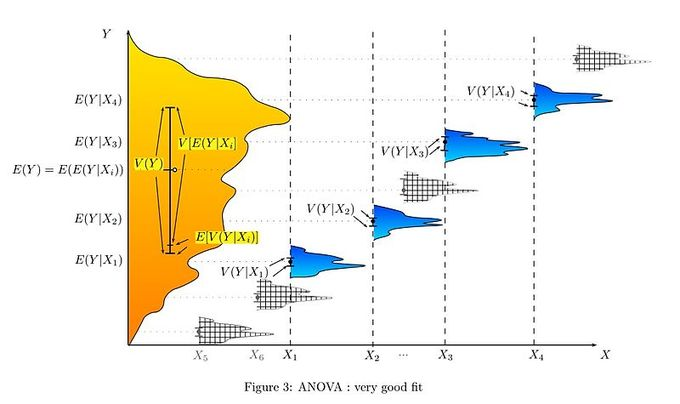ANOVA With Very Good Fit: This graph is a representation of a situation with a very good fit in terms of ANOVA statistics

## ANOVA Design

Many statisticians base ANOVA on the design of the experiment, especially on the protocol that specifies the random assignment of treatments to subjects.

### Learning Objectives

Differentiate one-way, factorial, repeated measures, and multivariate ANOVA experimental designs; single and multiple factor ANOVA tests; fixed-effect, random-effect and mixed-effect models

### Key Takeaways

#### Key Points

• Some popular experimental designs use one-way ANOVA, factorial ANOVA, repeated measures ANOVA, or MANOVA (multivariate analysis of variance ).
• ANOVA can be performed for a single factor or multiple factors.
• The classes of models use in ANOVA are fixed-effects models, random-effects models, and multi-effects models.

#### Key Terms

• ANOVA: Analysis of variance—a collection of statistical models used to analyze the differences between group means and their associated procedures (such as “variation” among and between groups).
• blocking: A schedule for conducting treatment combinations in an experimental study such that any effects on the experimental results due to a known change in raw materials, operators, machines, etc., become concentrated in the levels of the blocking variable.

There are several types of ANOVA. Many statisticians base ANOVA on the design of the experiment, especially on the protocol that specifies the random assignment of treatments to subjects. The protocol’s description of the assignment mechanism should include a specification of the structure of the treatments and of any blocking. It is also common to apply ANOVA to observational data using an appropriate statistical model. Some popular designs use the following types of ANOVA.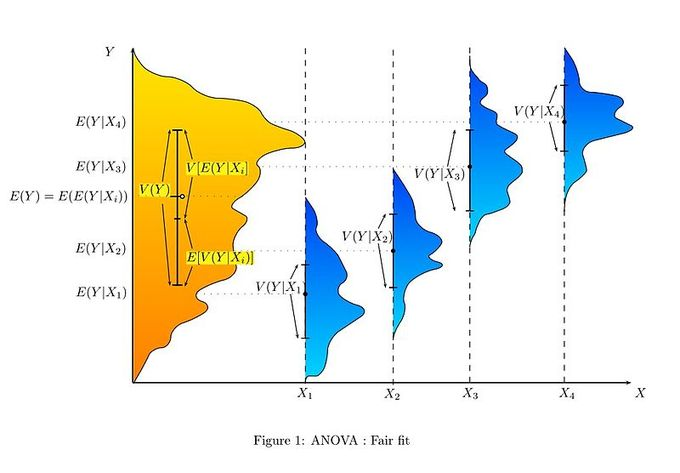ANOVA With Fair Fit: This graph shows a representation of a situation with a fair fit in terms of ANOVA statistics.

1. One-way ANOVA is used to test for differences among two or more independent groups. Typically, however, the one-way ANOVA is used to test for differences among at least three groups, since the two-group case can be covered by a $\text{t}$-test. When there are only two means to compare, the $\text{t}$-test and the ANOVA $\text{F}$-test are equivalent.
2. Factorial ANOVA is used when the experimenter wants to study the interaction effects among the treatments.
3. Repeated measures ANOVA is used when the same subjects are used for each treatment (e.g., in a longitudinal study).
4. Multivariate analysis of variance (MANOVA) is used when there is more than one response variable.

### ANOVA for a Single Factor

The simplest experiment suitable for ANOVA analysis is the completely randomized experiment with a single factor. More complex experiments with a single factor involve constraints on randomization and include completely randomized blocks. The more complex experiments share many of the complexities of multiple factors.

### ANOVA for Multiple Factors

ANOVA generalizes to the study of the effects of multiple factors. When the experiment includes observations at all combinations of levels of each factor, it is termed factorial. Factorial experiments are more efficient than a series of single factor experiments, and the efficiency grows as the number of factors increases. Consequently, factorial designs are heavily used.

The use of ANOVA to study the effects of multiple factors has a complication. In a 3-way ANOVA with factors $\text{x}$, $\text{y}$, and $\text{z}$, the ANOVA model includes terms for the main effects ($\text{x}$, $\text{y}$, $\text{z}$) and terms for interactions ($\text{xy}$, $\text{xz}$, $\text{yz}$, $\text{xyz}$). All terms require hypothesis tests. The proliferation of interaction terms increases the risk that some hypothesis test will produce a false positive by chance. Fortunately, experience says that high order interactions are rare. The ability to detect interactions is a major advantage of multiple factor ANOVA. Testing one factor at a time hides interactions, but produces apparently inconsistent experimental results.

### Classes of Models

There are three classes of models used in the analysis of variance, and these are outlined here.

### Fixed-Effects Models

The fixed-effects model of analysis of variance applies to situations in which the experimenter applies one or more treatments to the subjects of the experiment to see if the response variable values change. This allows the experimenter to estimate the ranges of response variable values that the treatment would generate in the population as a whole.

### Random-Effects Models

Random effects models are used when the treatments are not fixed. This occurs when the various factor levels are sampled from a larger population. Because the levels themselves are random variables, some assumptions and the method of contrasting the treatments (a multi-variable generalization of simple differences) differ from the fixed-effects model.

### Mixed-Effects Models

A mixed-effects model contains experimental factors of both fixed and random-effects types, with appropriately different interpretations and analysis for the two types. For example, teaching experiments could be performed by a university department to find a good introductory textbook, with each text considered a treatment. The fixed-effects model would compare a list of candidate texts. The random-effects model would determine whether important differences exist among a list of randomly selected texts. The mixed-effects model would compare the (fixed) incumbent texts to randomly selected alternatives.

## ANOVA Assumptions

The results of a one-way ANOVA can be considered reliable as long as certain assumptions are met.

### Learning Objectives

List the assumptions made in a one-way ANOVA and understand the implications of unit-treatment additivity

### Key Takeaways

#### Key Points

• Response variables are normally distributed (or approximately normally distributed).
• Samples are independent.
• Variances of populations are equal.
• Responses for a given group are independent and identically distributed normal random variables—not a simple random sample (SRS).
• The randomization-based analysis assumes only the homogeneity of the variances of the residuals (as a consequence of unit-treatment additivity ) and uses the randomization procedure of the experiment.

#### Key Terms

• ANOVA: Analysis of variance—a collection of statistical models used to analyze the differences between group means and their associated procedures (such as “variation” among and between groups).
• unit-treatment additivity: An assumption that states that the observed response from the experimental unit when receiving treatment can be written as the sum of the unit’s response $\text{y}_\text{i}$ and the treatment-effect $\text{t}_\text{j}$.
• simple random sample: A sample in which each individual is chosen randomly and entirely by chance, such that each individual has the same probability of being chosen at any stage during the sampling process, and each subset of $\text{k}$ individuals has the same probability of being chosen for the sample as any other subset of $\text{k}$ individuals.

The results of a one-way ANOVA can be considered reliable as long as the following assumptions are met:

• Response variables are normally distributed (or approximately normally distributed).
• Samples are independent.
• Variances of populations are equal.
• Responses for a given group are independent and identically distributed normal random variables—not a simple random sample (SRS).

Necessary assumptions for randomization-based analysis are as follows.

### Randomization-Based Analysis

In a randomized controlled experiment, the treatments are randomly assigned to experimental units, following the experimental protocol. This randomization is objective and declared before the experiment is carried out. The objective random-assignment is used to test the significance of the null hypothesis, following the ideas of C.S. Peirce and Ronald A. Fisher. This design-based analysis was developed by Francis J. Anscombe at Rothamsted Experimental Station and by Oscar Kempthorne at Iowa State University. Kempthorne and his students make an assumption of unit-treatment additivity.

In its simplest form, the assumption of unit-treatment additivity states that the observed response from the experimental unit when receiving treatment can be written as the sum of the unit’s response $\text{y}_\text{i}$ and the treatment-effect $\text{t}_\text{j}$, or
$\text{y}_{\text{i}, \text{j}} = \text{y}_\text{i}+\text{t}_\text{j}$
The assumption of unit-treatment additivity implies that for every treatment $\text{j}$, the $\text{j}$th treatment has exactly the same effect $\text{t}_\text{j}$ on every experiment unit. The assumption of unit-treatment additivity usually cannot be directly falsified; however, many consequences of unit-treatment additivity can be falsified. For a randomized experiment, the assumption of unit-treatment additivity implies that the variance is constant for all treatments. Therefore, by contraposition, a necessary condition for unit-treatment additivity is that the variance is constant. The use of unit-treatment additivity and randomization is similar to the design-based inference that is standard in finite-population survey sampling.